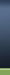HOME BZU Mail Box Online Games Radio and TV Cricket All Albums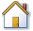BZU PAGES: Find Presentations, Reports, Student's Assignments and Daily Discussion; Bahauddin Zakariya University MultanProgram to find the sum of each row & column of a matrix of size n x m and if matrix is square, find the sum of the diagonals
 User Name Remember Me? Password#1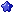bonfire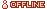M.Arsalan Qureshi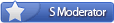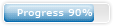Join Date: Oct 2008 Location: Garden Town, Multan Cantt Posts: 616 Program / Discipline: BSTS Class Roll Number: 09-31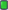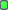Program to find the sum of each row & column of a matrix of size n x m and if matrix is square, find the sum of the diagonals

Program to find the sum of each row & column of a matrix of size n x m and
if matrix is square, find the sum of the diagonals also.

#include <iostream.h>
#include <conio.h>
int main()
{
clrscr();
int A,m,n,x,y,sum=0;
//Create a Matrix A
cout << "Enter number of rows and columns in Matrix A : \n";
cin>>n>>m;
cout << "Enter elements of Matrix A : \n";
for(x=1;x<n+1;++x)
for(y=1;y<m+1;++y)
cin>>A[x][y];
//Find sum of each row
for(x=1;x<n+1;++x)
{
A[x][m+1]=0;
for(y=1;y<m+1;++y)
A[x][m+1]=A[x][m+1]+A[x][y];
}
//Find sum of each column
for(y=1;y<m+1;++y)
{
A[n+1][y]=0;
for(x=1;x<n+1;++x)
A[n+1][y]+=A[x][y];
}
cout << "\nMatrix A, Row Sum (Last Column)" << " and Column Sum (Last Row) : \n";
for(x=1;x<n+1;++x)
{
for(y=1;y<m+2;++y)
cout << A[x][y] << " ";
cout << "\n";
}
//Print sum of each column
x=n+1;
for(y=1;y<m+1;++y)
cout << A[x][y] << " ";
cout << "\n";
if(m==n)
{
for(x=1;x<m+1;x++)
for(y=1;y<n+1;y++)
if(x==y)
sum+=A[x][y];
else
if(y==m-(x+1))
sum+=A[x][y];
}
cout << "Sum of diagonal elements is : " << sum << endl;
getch();
return 0;
}
This program takes in the number of rows (n) and columns (m) as well as the elements as a screen input in a matrix n x m.
It then calculates the sum of each row and each column and outputs it using the 'cout' command.
Also, if it is a square matrix, it calculates the sum of diagonal elements and prints it out.

Sample Input
3 3
9 8 7 6 5 4 3 2 1
Sample Output
Matrix A, Row Sum(Last Column) and Column Sum(Last Row) :
9 8 7 24
6 5 4 15
3 2 1 6
18 15 12
Sum of diagonal elements is : 15

Attached Files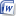Program to find the sum of each row & column of a matrix of size n x m and(www.bzupages.com).docx (12.7 KB, 170 views)
__________________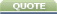Tags column, diagonals, find, matrix, program, row, size, square, sumCurrently Active Users Viewing This Thread: 1 (0 members and 1 guests)
 Thread Tools Search this ThreadShow Printable VersionEmail this Page Search this Thread: Advanced Search Display Modes Rate This ThreadLinear ModeSwitch to Hybrid ModeSwitch to Threaded Mode Rate This Thread: 5 : Excellent 4 : Good 3 : Average 2 : Bad 1 : Terrible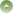Posting Rules You may not post new threads You may not post replies You may not post attachments You may not edit your posts BB code is On Smilies are On [IMG] code is On HTML code is OffTrackbacks are On Pingbacks are On Refbacks are On Forum Rules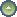Similar Threads Thread Thread Starter Forum Replies Last Post bonfire Object Oriented Programming 0 09-03-2011 12:26 AM bonfire Object Oriented Programming 0 01-03-2011 03:08 PM taha khan Visual Programming 0 10-12-2009 11:15 PM taha khan Visual Programming 0 10-12-2009 11:12 PM shmsa Visual Programming 0 06-12-2009 04:56 PM

Best view in Firefox
Almuslimeen.info | BZU Multan | Dedicated server hosting
Note: All trademarks and copyrights held by respective owners. We will take action against any copyright violation if it is proved to us.

All times are GMT +5. The time now is 02:52 PM.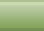Contact Us - BZU Multan - Archive - Top